PROBLEM #1, SET 3 - NUMBERS and MATH

1. How many ounces is 45.0 tonnes?

This problem is clearly a DA - only problem because it asks you to convert from metric tonnes to English ounces. Here is a list of the definitions to use:

1 tonne = E3 kg
1 kg = E3 g
1 # = 453.6 g (the metric - to - English "bridge")
OR 1kg = 2.2 # (This definition only has two significant figures.)
and 1 # = 16 Oz

As for any DA problem, begin with what you know and use the definitions as conversion factors so you can cancel the units you don't want and leave the units you do. Actually write down the definitions as you see here until you become more proficient with their use.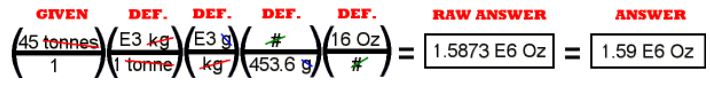The calculator math is:   45 x 1000 x 1000 Ã· 453.6 x 16 =

The raw answer is the number taken directly off of the calculator and the correct units. The final answer is given here shortened to the correct number of significant figures, three in this case. Your calculator will show you large numbers in the scientific notation style.

The answer comes out only slightly different if you use the definition, "1 kg = 2.2 #" rather than going from kilograms to grams to pounds. You try it for yourself.

PROBLEM #2, SET 3 - NUMBERS and MATH

2. How many liters are in 8.55 cubic miles of ocean water?

This problem is a DA - only problem because it asks you to convert from one unit of volume to another, cubic miles to liters. Cubic mile is an English unit and liter is a metric unit. There are at least two good ways do this problem with the information you have: cubic miles to cubic feet to cubic inches to cubic centimeters to milliliters to liters, or cubic miles to cubic feet to gallons to quarts to liters. The first sequence is more accurate because the definitions from cubic feet to gallons and from quarts to liters are not exact.

As for any DA problem, begin with what you know and use the definitions as conversion factors so you can cancel the units you don't want and leave the units you do.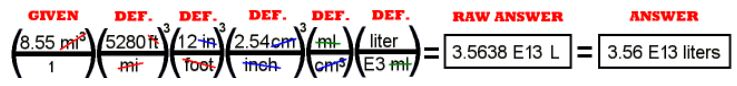Notice the way to use linear definitions like 1 in = 2.54 cm CUBED to make them serve for DA changes in volume. This is a demonstration of how powerful the DA system is with just a few definitions.

The math is done by multiplying everything on top (in the numerator) and dividing by everything on the bottom (in the denominator).
Here is the calculator math: 8.55 x 5280 x 5280 x 5280 x 12 x 12 x 12 x 2.54 x 2.54 x 2.54 Ã· 1000 =

The raw answer is the number taken directly off of the calculator and the correct units. The final answer is given here shortened to three significant figures. Your calculator will show you large numbers in the scientific notation style.

PROBLEM #3, SET 3 - NUMBERS and MATH

3. Water has a density of one gram (1.00g) per cubic centimeter. What is that in pounds per gallon?

This problem is a DA - only problem beacuse it asks you to convert from one unit of density to another, grams per cc to pounds per gallon. The problem is much easier if you think of changing grams to pounds and cubic centimeters to gallons. Again there are several ways to do this.

1 kg = 2.2 #
1 gal = 4 qt
1 liter = 1.06 qt
1 cc = 1 ml

As for any DA problem, begin with what you know and use the definitions as conversion factors so you can cancel the units you don't want and leave the units you do.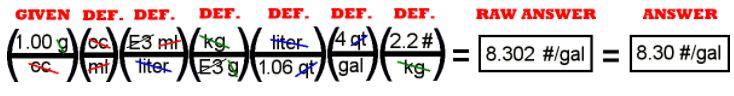The math is done by multiplying everything on top (in the numerator) and dividing by everything on the bottom (in the denominator).
Here is the calculator math: 1 Ã· 1.06 x 4 x 2.2 =

Notice that it is best to start the calculator math with a "1" to avoid a problem with having the first number in the numerator. The raw answer is the number taken directly off of the calculator and the correct units. The final answer is given here shortened to three significant figures.

As with the calculation in #1 in this series, you could use 1 kg = 1000g and 1 # = 453.6 g instead of 1 kg = 2.2 #. The first sequence is good for four significant figures, while the single definition is only good for two significant figures.

The number of about 8.3 pounds per gallon of water is a good one to remember as long as the US is going to insist upon using the unit of gallons. Many of the common materials we encounter, particularly in a grocery store, have densities in the vicinity of the density of water. At one time (when the British Empire could brag that the sun never set on it), there was a useful rule of thumb, "A pint is a pound the world 'round." That is about right for any material close to the density of water. One gallon is eight pints and one gallon of water has a weight of 8.3 pounds.

PROBLEM #4, SET 3 - NUMBERS and MATH

4. A roadsign posts a 50.0 MPH limit. What is that in KPH?

This problem is a DA - only problem beacuse it asks you to convert from one unit of velocity to another, miles per hour to kilometers per hour. The problem is much easier if you think of changing just the miles to kilometers. The hour unit in the denominator does not need changing.

1 mile = 5280 ft
1 ft = 12 inches
1 inch = 2.54 cm   (English - to - metric "bridge")
1 km = E5 cm

As for any DA problem, begin with what you know and use the definitions as conversion factors so you can cancel the units you don't want and leave the units you do.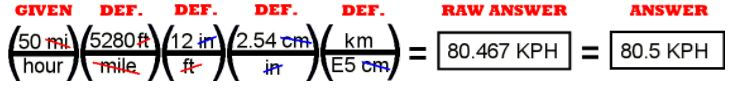The math is done by multiplying everything on top (in the numerator) and dividing by everything on the bottom (in the denominator).
Here is the calculator math: 50 x 5280 x 12 x 2.54 Ã· 1 E 5 =

As in the calculations above, the math can be done staight across withoug stopping. If you stop to gather the numbers on top and on bottom and then do the division, you risk losing some numbers.

The raw answer is the number taken directly off of the calculator and the correct units. The final answer is given here shortened to three significant figures. You can easily check this conversion by the speedometer of any recent model car. The MPH and KPH are displayed together on the speedometer readout. Look for yourself. Is 50 MPH about equal to 80 KPH?

PROBLEM #5, SET 3 - NUMBERS and MATH

5. How large in square feet is a 525 square meter house?

This problem is a DA - only problem beacuse it asks you to convert from one unit of area to another, square meters to square feet. It is easy to think of this problem in terms of going from meters to centimeters to inches to feet and squaring each step.

1 ft = 12 inches
1 inch = 2.54 cm   (the metric - to - English "bridge")
1 m = E2 cm

As for any DA problem, begin with what you know and use the definitions as conversion factors so you can cancel the units you don't want and leave the units you do.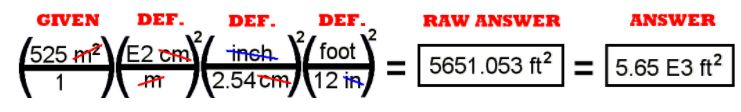The math is done by multiplying everything on top (in the numerator) and dividing by everything on the bottom (in the denominator).,
Here is the calculator math: 525 x 100 x 100 Ã· 2.54 Ã· 2.54 Ã· 12 Ã· 12 =

The raw answer is the number taken directly off of the calculator and the correct units. The final answer is given here shortened to three significant figures and expressed in scientific notation.

PROBLEM #6, SET 3 - NUMBERS and MATH

6. How many square yards of paint are needed on the ceiling of a room that is 24.0 feet wide, 51.0 feet long and ten feet high?

This problem is likely best done with W5P method because it asks for an area when you are given two lengths. The units are all English system.

GIVEN:   l1 = 24.0 ft,     l2 = 51.0 ft,     l3 = 10.0 ft, (BUT NOT NEEDED)
You know this from the units and dimensions table in the Units section of Lessons.

FIND:   an area, A
The question asks for an area, A in square yards. You know the symbol for area from the units and dimensions table in the Units section of Lessons.

FORMULA:   A = l l
You know two lengths. You are asked to find an area. The formulas come from the formula section of Units in Lessons.

SOLVE: Solve for one of the lengths. Substitute the known values from GIVEN into the working equation, simplify, and do the math. Here the math includes some DA to get the units right.

WHAT YOU WRITE DOWN SHOULD LOOK LIKE THIS:

GIVEN:   l1 = 24.0 ft,     l2 = 51.0 ft,

FIND:   an area, A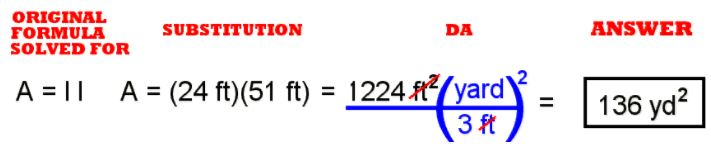Notice the neat way that the DA was included in the problem. (See the math work above in the blue parentheses.) The definition "1 yard = 3 feet" was used twice (squared) to get the answer into square yards.

The calculator math is:   24 x 51 Ã· 3 Ã· 3 =

ANSWER: Calculate the numerical answer and show your cancellation of the units. The raw answer does not need to be changed into scientific notation or shortened to any fewer significant digits.

PROBLEM #7, SET 3 - NUMBERS and MATH

7. Gasoline has a density of 5.83 pounds per gallon. What volume tank would you need to store three tons of gasoline?

This problem is likely best done with W5P method because it asks for a volume when you are given a density and a weight. The units are all English system. Notice that the formula has to be for the English system weight density.

GIVEN:   Dw = 5.83 #/gal,     Fw = 3.00 tons
You know this from the units and dimensions table in the Units section of Lessons.

FIND:   a volume, V
The question asks for a volume, V. You know the symbol for volume from the units and dimensions table in the Units section of Lessons.

FORMULA:   D = Fw/V
You know the weight and the density. You are asked to find a volume. The formulas come from the formula section of Units in Lessons.

SOLVE: Solve for the volume. Substitute the known values from GIVEN into the working equation, simplify, and do the math. Here the math includes some DA to get the units right.

WHAT YOU WRITE DOWN SHOULD LOOK LIKE THIS:

GIVEN:   Dw = 12.0 #/gal,     Fw = 17.0 tons

FIND:   a volume, V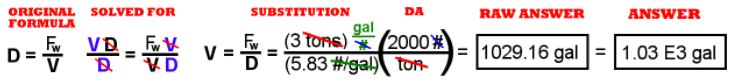The calculator math is:   3 Ã· 5.83 x 2000 =

ANSWER: Notice the way the units of density in the denominator were handled. The complex units presenting a fraction in the denominator can be simplified by inverting and placing in the numerator. The same set of units could be represented by the gallon unit in the numerator and the pound unit in the denominator. All of these are the same, but the less complicated the units appear, the less likely you are to make a mistake as you arrange the problem. Calculate the numerical answer, show your cancellation of the units, and show the raw answer (the units that remain and the number on your calculator). Change the raw answer to the correct number of significant digits and change into scientific notation if the answer is greater than 1000 or less than 1/1000.

PROBLEM #8, SET 3 - NUMBERS and MATH

8. The bathtub in the residential suite of the White House had to be enlarged for Warren G. Harding. He weighed 370 pounds. (Burp.) The bathtub is four feet wide, six feet long and three feet high. How many gallons of water are needed to fill the WGH Memorial Bathtub? (1 gal = 231 cu.in.) (Consider all numbers to have three significant figures here.)

This problem is likely best done with W5P method because it asks for a volume (in gallons) when you are given three lengths. The units are all English system.

GIVEN:   l1 = 4.00 ft,     l2 = 6.00 ft,     l3 = 3.00 ft,
You know this from the units and dimensions table in the Units section of Lessons.

We could list Warren G. Harding's weight here as part of the given material, but the equation does not need that information, so we would do just as well to leave it out. The point of that is that in "textbook" problems, you usually use all the data given. That just does not happen in real life. In live sidutations, if you want an answer, you have to know what data and what formulas to use.

FIND:   a volume, V
The question asks for a volume, V. You know the symbol for volume from the units and dimensions table in the Units section of Lessons.

FORMULA:   V = l l l    You know three lengths. You are asked to find a volume. The formulas come from the formula section of Units in Lessons.

SOLVE: The known and memorized formula is already solved for the volume. Substitute the known values from GIVEN into the working equation, simplify, and do the math. Here the math includes some DA to get the units right.

WHAT YOU WRITE DOWN SHOULD LOOK LIKE THIS:

GIVEN:   l1 = 4.00 ft,     l2 = 6.00 ft,     l3 = 3.00 ft,

FIND:   a volume, V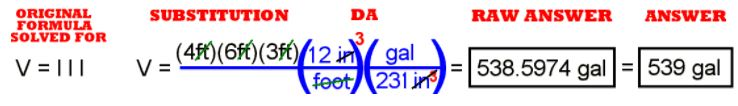The calculator math is:   4 x 6 x 3 x 12 x 12 x 12 Ã· 231 =

ANSWER: Calculate the numerical answer, show your cancellation of the units, and show the raw answer (the units that remain and the number on your calculator). Change the raw answer to the correct number of significant digits and change into scientific notation if the answer is greater than 1000 or less than 1/1000. The raw answer does not need to be in scientific notation, but must be rounded to three significant digits.

PROBLEM #9, SET 3 - NUMBERS and MATH

9. The density of water is 8.34 #/gal. What weight of water is needed to fill the WGH Memorial Bathtub?

This problem is likely best done with W5P method because it asks for a weight when you are given an English weight density and a volume from the previous question. The units are all English system.

GIVEN:   Dw = 8.34 #/gal,     V = 538.6 gal (from #8)
You know this from the units and dimensions table in the Units section of Lessons.

FIND:   a weight, Fw
The question asks for a weight, Fw. You know the symbol for weight from the units and dimensions table in the Units section of Lessons.

FORMULA:   Dw = Fw/V
You know a weight density and a volume. You are asked to find a weight. The formulas come from the formula section of Units in Lessons.

SOLVE: Solve for the weight. Substitute the known values from GIVEN into the working equation, simplify, and do the math. Here the math includes some DA to get the units right.

WHAT YOU WRITE DOWN SHOULD LOOK LIKE THIS:

GIVEN:   Dw = 8.34 #/gal,     V = 538.6 gallons

FIND:   a weight, Fw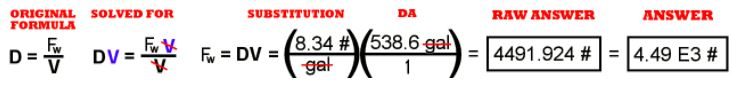The calculator math is:   8.34 x 538.6 Ã· 12 Ã· 17 x 12 =   or just:   51 Ã· 17 =

ANSWER: Calculate the numerical answer, show your cancellation of the units, and show the raw answer (the units that remain and the number on your calculator). Change the raw answer to the correct number of significant digits and change into scientific notation if the answer is greater than 1000 or less than 1/1000.

PROBLEM #10, SET 3 - NUMBERS and MATH

10. Mercury is 13.6 kg/liter. What mass of mercury is needed to fill the WGH Memorial Bathtub?

This problem is likely best done with W5P method because it asks for a mass when you are given a density and a volume (from #8 again). The units of mass and density are metric, but you know only an English volume for the bathtub.

GIVEN:   D = 13.6 kg/liter,     V = 53.86 gal
You know this from the units and dimensions table in the Units section of Lessons.

FIND:   a mass, m
The question asks for a mass, m. You know the symbol for mass from the units and dimensions table in the Units section of Lessons.

FORMULA:   D = m/V
You know a volume and density. You are asked to find a mass. The formulas come from the formula section of Units in Lessons.

SOLVE: Solve for the mass. Substitute the known values from GIVEN into the working equation, simplify, and do the math. Here the math includes some DA to get the units right.

WHAT YOU WRITE DOWN SHOULD LOOK LIKE THIS:

GIVEN:   D = 13.6 kg/liter,     V = 538.6 gal

FIND:   a mass, m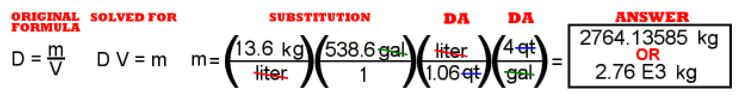The calculator math is:   13.6 x 536.8 Ã· 1.06 x 4 =

ANSWER: Calculate the numerical answer, show your cancellation of the units, and show the raw answer (the units that remain and the number on your calculator). Change the raw answer to the correct number of significant digits and change into scientific notation if the answer is greater than 1000 or less than 1/1000.

PROBLEM #11, SET 3 - NUMBERS and MATH

11. The velocity of light is 186,000 mi/sec. It takes radio signals 17.6 minutes to go from Earth to Jupiter at their closest approach. How far apart are the two planets at that time?

This problem is likely best done with W5P method because it asks for a distance when you are given a rate (or velocity) and a time. The units are English system from the miles. The time units are both metric and English.

GIVEN:   v = 186,000 miles,     t = 17.6 minutes
You know this from the units and dimensions table in the Units section of Lessons. Notice that the "v" for velocity is the lower case "v."

FIND:   a length, d
The question asks for a length or distance, d. You know the symbol for length from the units and dimensions table in the Units section of Lessons. The symbol "d" is used for distance because the rate equation uses that.

FORMULA:   v t = d
You know a velocity and a time. You are asked to find a distance. The formulas come from the formula section of Units in Lessons.

SOLVE: The distance is already solved for in the known equation. Substitute the known values from GIVEN into the working equation, simplify, and do the math. Here the math includes some DA to get the units right.

WHAT YOU WRITE DOWN SHOULD LOOK LIKE THIS:

GIVEN:   v = 186,000 miles/second,     t= 17.6 minutes

FIND:   a length, d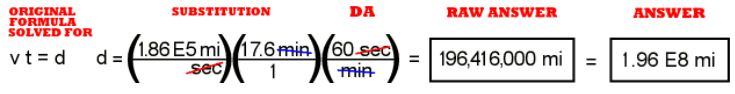The calculator math is: 1.86 E 5 x 17.6 x 60 =

ANSWER: Calculate the numerical answer, show your cancellation of the units, and show the raw answer (the units that remain and the number on your calculator). Change the raw answer to the correct number of significant digits and change into scientific notation if the answer is greater than 1000 or less than 1/1000.

PROBLEM #12, SET 3 - NUMBERS and MATH

12. A car takes on 22.7 L of gasoline. How many Floz is that?

Remember that a fluid ounce (Floz) is a unit of volume whereas an ounce (Oz) is a unit of force or weight.

This problem is clearly a DA - only problem because it asks you to convert from liters, a metric unit of volume to fluid ounces, an English unit of volume. Here is a list of the definitions to use:

1 liter = 1.06 quarts (the bridge to the English system in volume)
1 quart = 2 pints
1 cup = 8 Floz

As for any DA problem, begin with what you know and use the definitions as conversion factors so you can cancel the units you don't want and leave the units you do.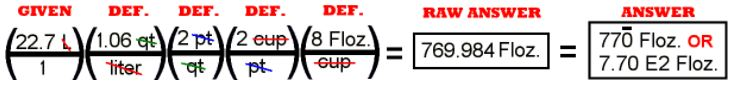The calculator math is: 22.7 x 1.06 x 2 x 2 x 8 =

The treatment of the answer in this case is interesting. The number is actually too small to need to be written in scientific notation. (It is less than 1000.) But the number "770" just as it is suggests only two significant digits. There are two ways to make sure the three significant digits are recognized. Writing the number in scientific notation at the "E2" level or placing a bar over the training zero will show that the third digit, the zero, is significant.

PROBLEM #13, SET 3 - NUMBERS and MATH

13. Osmium metal is 22.0 g/mL. What is the volume of 2.50 kg of it?

If you recognize "22.0 g/mL" as a density, you know your units. If you don't notice it as a density, you might have some trouble knowing what to do with the entire problem. Once you see that, the problem is easiest done with W5P method because it asks for a volume when you are given a density and a mass. The units are all metric.

GIVEN:   D= 22.0 g/mL     m = 2.50 kg
You know this from the units and dimensions table in the Units section of Lessons.

FIND:   a volume, V
The question asks for a volume, V. You know the symbol for volume from the units and dimensions table in the Units section of Lessons.

FORMULA:   D = m/V
You know a density and a mass. You are asked to find a volume. The formulas come from the formula section of Units in Lessons.

SOLVE: Solve for the volume. Substitute the known values from GIVEN into the working equation, simplify, and do the math. Here the math includes some DA to get the units right.

WHAT YOU WRITE DOWN SHOULD LOOK LIKE THIS:

GIVEN:   D = 22.0 g/mL     m = 2.50 kg

FIND:   a volume, V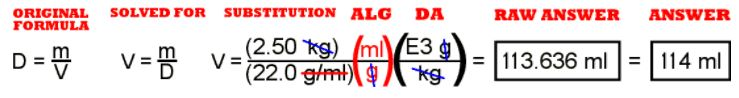The calculator math is:   2.5 Ã· 22 x 1000 =

ANSWER: Calculate the numerical answer, show your cancellation of the units, and show the raw answer (the units that remain and the number on your calculator). Change the raw answer to the correct number of significant digits and change into scientific notation if the answer is greater than 1000 or less than 1/1000.

PROBLEM #14, SET 3 - NUMBERS and MATH

14. Concentrated sulfuric acid is 1.84 kg/L. What is the mass of 500 mL of it?

Again you need to see that 1.84 Kg/L is a density. Once you know that you have a density and a volume and need a mass, the problem seems a lot easier. W5P can be used here. The units are all metric.

GIVEN:   V = 500 mL     D = 1.84 kg/L
You know this from the units and dimensions table in the Units section of Lessons.

FIND:   a mass, m
The question asks for a mass, m. You know the symbol for mass from the units and dimensions table in the Units section of Lessons.

FORMULA:   D = m/V
You know a density and a volume. You are asked to find a mass. The formulas come from the formula section of Units in Lessons.

SOLVE: Solve for the mass. Substitute the known values from GIVEN into the working equation, simplify, and do the math. Here the math includes some DA to get the units right.

WHAT YOU WRITE DOWN SHOULD LOOK LIKE THIS:

GIVEN:   V = 500 mL     D = 1.84 kg/L

FIND:   a mass, m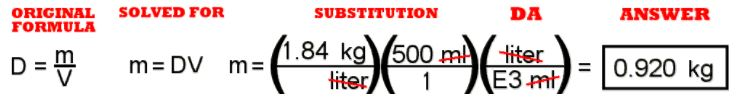The calculator math is:   1.84 x 500 Ã· 1000 =

ANSWER: Calculate the numerical answer, show your cancellation of the units, and show the raw answer (the units that remain and the number on your calculator). Change the raw answer to the correct number of significant digits and change into scientific notation if the answer is greater than 1000 or less than 1/1000. No change from the raw answer is needed here if you leave it in units of kg.

PROBLEM #15, SET 3 - NUMBERS and MATH

15. A man is 5 ft. 11 inches tall. How many meters is that?

This problem is clearly a DA - only problem because it asks you to convert from feet and inches to meters. Notice the English measurement of length is a mixed number, that is, the distance is "5 feet plus eleven inches." In order to use this in the DA type of math, it will be necessary to convert it to either feet or inches. It is often useful to think in DA even for such a small operation.

5 ft (12 in/ft) = 60 inches and add the 11 inches to it to make 71 inches.

Here is a list of the definitions to use:
1 inch = 2.54 cm (the bridge from English to metric in length)
1 m = 100 cm

As for any DA problem, begin with what you know and use the definitions as conversion factors so you can cancel the units you don't want and leave the units you do.

>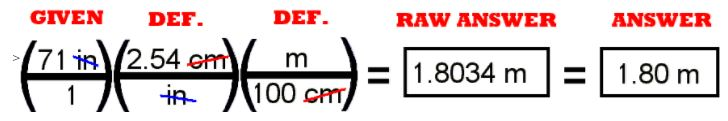The calculator math is:   71 x 2.54 Ã· 100 =

if (isMyPost) { }